HomeBlog

# Selection of Drive Resistor: MOSFET | Gate Drive

The larger the resistance of the drive, the longer the turn-on time of MOSFET, and the longer the voltage and current overlap time in the switching time, the greater the switching loss. Therefore, the smaller the resistance, the better the drive resistance should be, provided that the drive resistance can provide enough damping to prevent the drive-current oscillation.

When designing switch power supply or motor drive circuit with MOSFET, the factors such as on resistance, maximum voltage and maximum current of MOSFET should be considered. In general, the MOSFET tube can be divided into the enhanced and depleted, P-channel or N-channel is a total of 4 types, but the enhanced NMOS tube and PMOS tube are mainly used, in these two commonly mentioned enhanced type, the more commonly used is NMOS, The reason is its small on-resistance and easy to manufacture. However, it is not enough to consider these, because the current will have different losses in various devices, so we must ensure that sufficient current to drive the MOSFET.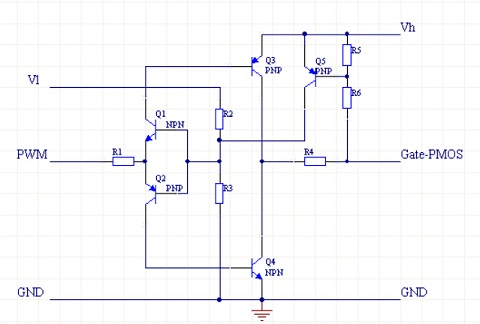Figure 1. MOS Schematic Diagram

In this paper, we will discuss the calculation of the MOS gate drive resistor. The range of the MOSFET drive resistance is between 5~100ohms, so how to further optimize the selection of the resistance value in this range?

Equivalent Drive Circuit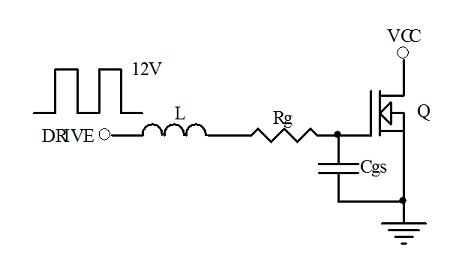Figure 2. Equivalent Drive Circuit

L is the PCB line inductor, according to the professional experience its straight line value is 1nH/ mm, considering other line factors, take L=Length +10 (nH), where Length unit is mm.

Rg is the gate drive resistance, and the driving signal is a square wave with a peak value of 12 V.

Cgs is the gate and source capacitance of MOSFET, with different tubes and driving voltage its value will be different, here is 1nF.

VL+VRg+VCgs=12V

Taking drive circuit: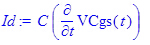Getting differential equation of driving voltage of Cgs:Obtaining Transformation function by method of Laplace transform: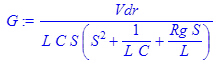This is a third-order system, which is an overdamped vibration when its poles are three different real roots, there are two same solid roots is critical damped vibrations, and there are imaginary roots is underdemped vibrations, which will generate waves of oscillation up and down at the gate of MOFET. This is something we do not want to see, so the choice of gate resistance Rg should make it work in the critical damping and over damping states, but the parameter error is actually working in the overdamped state.

Based on the above, therefore, the minimum range of Rg values can be obtained according to the length of the line.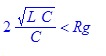Making the length of running line of 20mm and 70mm respectively: L20= 30nH , L70= 80nH, then Rg20=8.94Ω, Rg70=17.89Ω, Here are the voltage and current waveforms

Figure 3. Driving Voltage Ripple Curve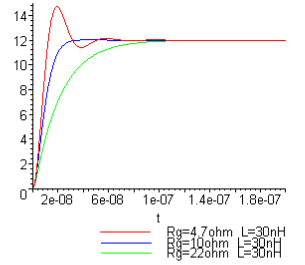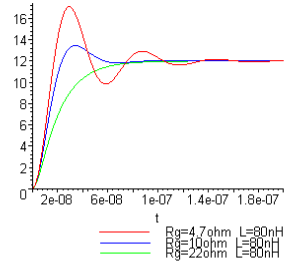Figure 4. Driving Current Ripple Curve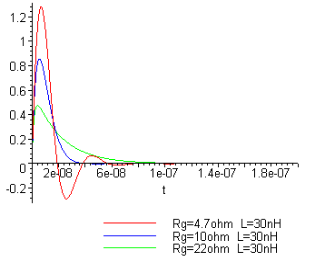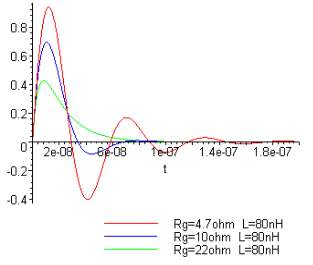According to the diagram when the Rg is small, the driving voltage surge will be higher, more and more oscillation will exist when the L becomes large, and the performance of MOSFET and other devices will be affected obviously. However, when the resistance value is too large, the driving waveform will rise slowly, while it will have a negative effect when the MOSFET has a large current passing through.

In addition, we should note that when L is small, the peak value of driving current is larger, and the output capacity of general IC is limited. When the actual driving current reaches the maximum value of IC output, the output of IC is equivalent to a constant current source. When Cgs is charged linearly, the rising of driving voltage waveform will slow down. The current curve may be shown on the follow (the inductance does not work because the current is constant), this may have an impact on the reliability of the IC, and a small step or burr may occur in the rise of the voltage waveform.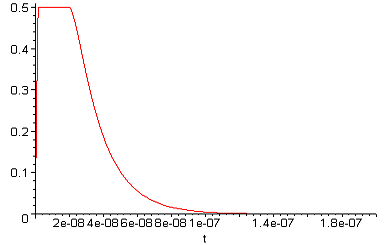Figure 5. Current Curve

The PWM OUT output of the general IC is shown in the left figure. The internal integration includes the current-limiting resistor Rsource and Rsink, usually Rsource > Rsink, but the actual values are related to the peak driving output ability of the IC. It can be approximately considered that R=Vcc/Ipeak. The drive output capacity of IC is about 0.5A, and meanwhile Rsource is about 20Ω.

From the previous voltage and current curves, we can see that the IC driver can drive MOSFET,  but the drive line is usually not a straight line, the inductance may be greater, and in order to prevent external interference, it is necessary to use the Rg drive resistor to suppress. This resistance should be as close as possible to the gate of the MOSFET when considering the effect of the line distribution capacitance.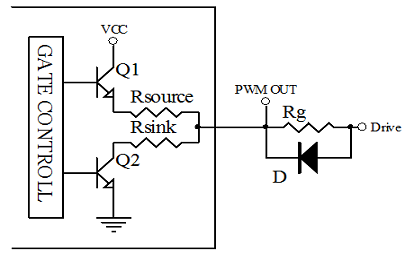Figure 6. PWM OUT

The effect of Rg and L on rising time: (Cgs=1nF, VCgs=0.9*Vdrive)

 TR(nS) 19 49 230 20 45 229 Rg(ohm) 10 22 100 10 22 100 L(nH) 30 30 30 80 80 80

It can be seen that L has little effect on TR, but Rg has great influence on TR. TR can be estimated approximately by 2*Rg*Cgs. Usually, the rise time is less than 20 percent of the conduction time, and the loss of the MOSFET switch when it is switched on will not cause a heat problem. So when the minimum conduction time of MOSFET is determined, the maximum value of Rg is determined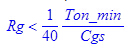. Generally, the smaller the Rg is, the better, but if considering the EMI, its value should be taken as large as possible.

The selection of resistor in MOSFET on-state is discussed above. In order to ensure the fast discharge of gate charge in MOSFET off-state, the resistance should be as small as possible, which is the reason of Rsink<Rsource. To ensure rapid discharge, a diode can be connected in parallel on the Rg. When the discharge resistance is too small, it will also cause resonance due to the inductance of the line (so in some applications there will be a small resistance on the diode.). But the reverse current of the diode is not conductive, at the same time, the Rg is involved in the reverse resonant circuit. Therefore, the peak of reverse resonance can be suppressed. This Diodes usually use a high frequency and small signal tube 1N4148.

In practice, we should also consider the influence of the gate and drain of MOSFET and a capacitor Cgd. When MOSFET is on, Rg has to charge Cgd, which will change the voltage rise slope. When off, VCC will charge Cgs through Cgd. In this case, the charge on Cgs must be removed quickly, otherwise, it will lead to abnormal conduction of MOSFET.Figure 7. MOSFET Schematic DiagramSearch
Inquiry
Order
Track

Delivery
FedEx
UPS
DHL
TNT

Payment Terms
By PayPal
By Credit Card
By Wire Transfer
By Western Union

After-sales Service
Quality Control
Guarantee
Return & Replacement